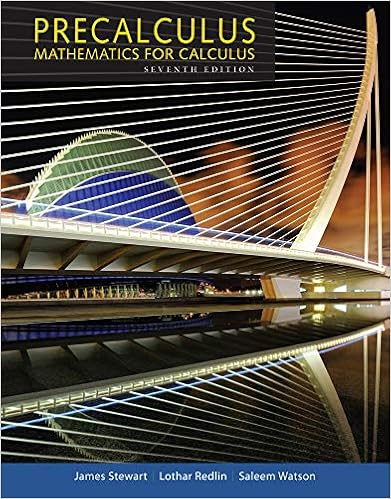Which of the following functions is neither even nor

• Test Prep
• 14
• 100% (7) 7 out of 7 people found this document helpful

This preview shows page 5 - 11 out of 14 pages.

We have textbook solutions for you!
The document you are viewing contains questions related to this textbook.The document you are viewing contains questions related to this textbook.
Chapter 9 / Exercise 3
Precalculus: Mathematics for Calculus
Redlin/StewartExpert Verified
10. Which of the following functions is neither even nor odd? a) f ( x ) = 4 x 2 – 3 x + 2 b) g ( x ) = 3 x 3 – 2 c) h ( x ) = 6 x 2 + 1 d) f ( x ) = 8 x 4 – 5 x
11. Find the magnitude of the resultant of two vectors u and v , given = u 5 , = v 4 and the angle between the vectors when they are arranged tail to tail is 65°.
12. The slope of the vector that is perpendicular to the scalar equation 2 x – 6 y + 1 = 0: 6 2 3
13. The dot product of a = (2, 5, 1) and b = (–5, 4, 2): 5
14. Given vectors = u ( (2, 4, –3) and = v ( (6, –2, –1), × u v is a) (–10, –16, –28) b) (12, –8, 3) c) (8, 2, –4) d) (2, –20, 20)
We have textbook solutions for you!
The document you are viewing contains questions related to this textbook.The document you are viewing contains questions related to this textbook.
Chapter 9 / Exercise 3
Precalculus: Mathematics for Calculus
Redlin/StewartExpert Verified
Practice Test Calculus and Vectors, MCV4U-B 15. Given two vectors u = (14, –6) and v = (–2, 5), the projection of u on v is
4 Part B: Knowledge and Understanding (16 marks) (approximate time: 15 minutes) 16. Find the equation of the tangent line to = + f x x x ( ) 2 4 7 3 at (2, 15). (4 marks)
Practice Test Calculus and Vectors, MCV4U-B 5 17. Determine the scalar, vector, and parametric equations of the plane that passes through the points P (1, 0, 4), Q (3, 1, –6) and R (–2, 3, 5). (12 marks) Part C: Thinking (22 marks) (approximate time: 30 minutes) 18. For the function = f x x x ( ) 2 2 , find the following: a) the x - and y -intercepts (2 marks) 4 2
Practice Test Calculus and Vectors, MCV4U-B 6 b) the horizontal and vertical asymptotes (2 marks) c) the first and second derivatives (6 marks: 3 marks each)
Practice Test Calculus and Vectors, MCV4U-B 7 d) any local maximum or minimum points (2 marks) e) the intervals of increasing and decreasing (3 marks)
Practice Test Calculus and Vectors, MCV4U-B 8
•••